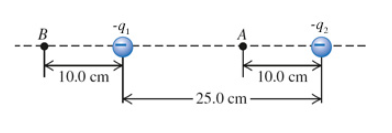# Problem: Two point charges are separated by 25.0 cm (see the figure (Figure 1) ). Assume that q1 = 6.05 nC and q2 = 11.5 nC.A. Find the magnitude of the net electric field these charges produce at point A.B. Find the magnitude of the net electric field these charges produce at point B.C. What would be the magnitude of the electric force this combination of charges would produce on a proton at A?

###### FREE Expert Solution

In this problem, we're going to use the equation for the electric field:

$\overline{){\mathbf{E}}{\mathbf{=}}\frac{\mathbf{k}\mathbf{q}}{{\mathbf{r}}^{\mathbf{2}}}}$, where k is Coulomb's constant, q is change, and r is the distance between the charge and the point where the electric field is produced.

The electric force is given by:

$\overline{){\mathbf{F}}{\mathbf{=}}{\mathbf{q}}{\mathbf{E}}}$.

A.

Electric field lines are directed toward negative point charges and away from positive charges.

At point A, the electric field due to q1 will be directed on the negative x-axis while the electric field due to q2 will be on the positive x-axis.

91% (352 ratings)###### Problem Details

Two point charges are separated by 25.0 cm (see the figure (Figure 1) ). Assume that q1 = 6.05 nC and q2 = 11.5 nC.A. Find the magnitude of the net electric field these charges produce at point A.

B. Find the magnitude of the net electric field these charges produce at point B.

C. What would be the magnitude of the electric force this combination of charges would produce on a proton at A?

Frequently Asked Questions

What scientific concept do you need to know in order to solve this problem?

Our tutors have indicated that to solve this problem you will need to apply the Electric Field concept. You can view video lessons to learn Electric Field. Or if you need more Electric Field practice, you can also practice Electric Field practice problems.

What professor is this problem relevant for?

Based on our data, we think this problem is relevant for Professor Velissaris' class at UCF.